# RD Sharma Solutions for Class 11 Chapter 19 - Arithmetic Progressions Exercise 19.2

In Exercise 19.2, we shall discuss problems based on the general terms of an A.P., which includes finding the nth term in the given expression. The solutions of the exercise wise problems under this chapter are prepared by experienced faculty team at BYJU’S after conducting vast research on each concept. The main aim of designing solutions is to help students with their board exam preparation and thereby, to secure good marks. On regular practice, students can achieve their goals. RD Sharma Class 11 Maths Solutions pdf are available here, students can make use of it by downloading from the links provided below.

## Download the pdf of RD Sharma Solutions for Class 11 Maths Exercise 19.2 Chapter 19 – Arithmetic Progressions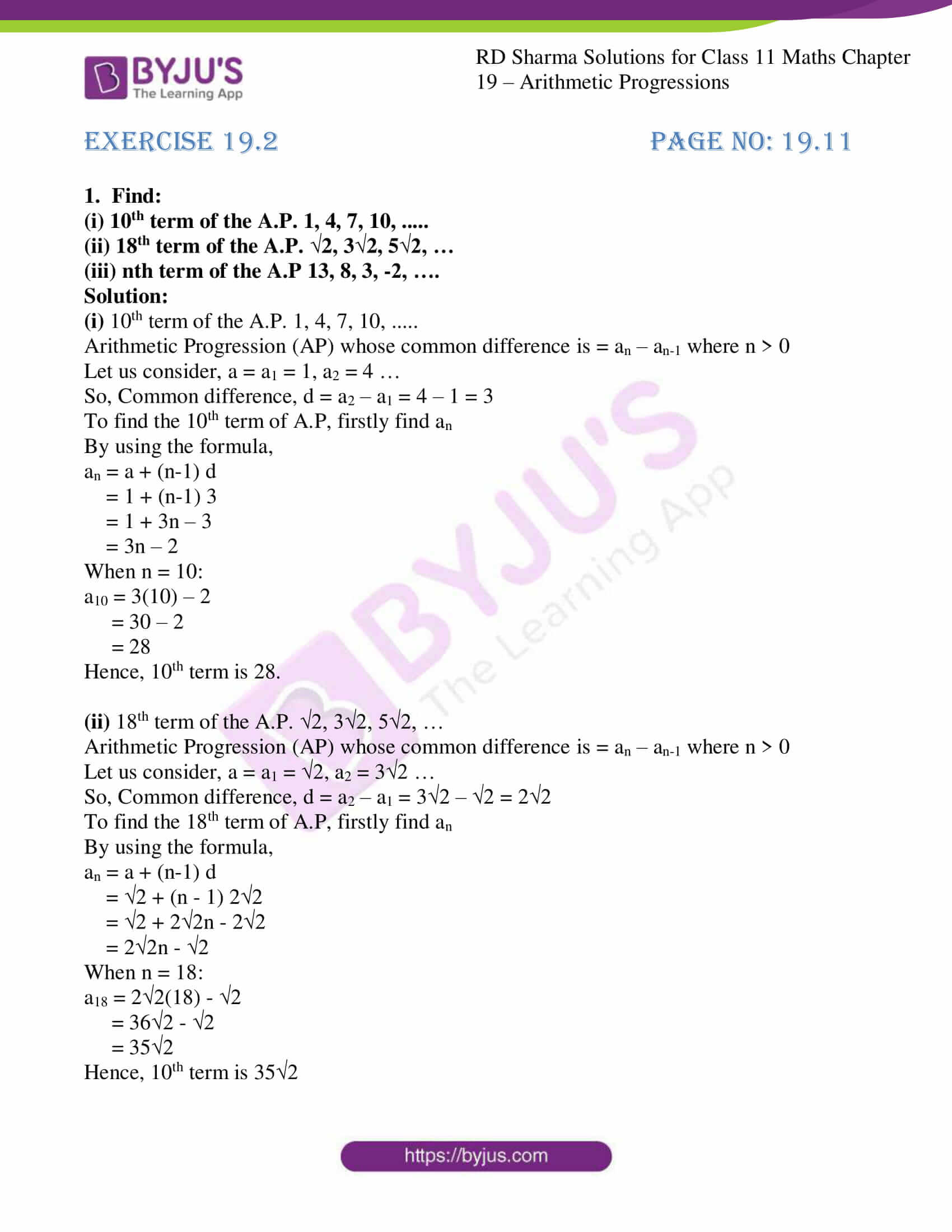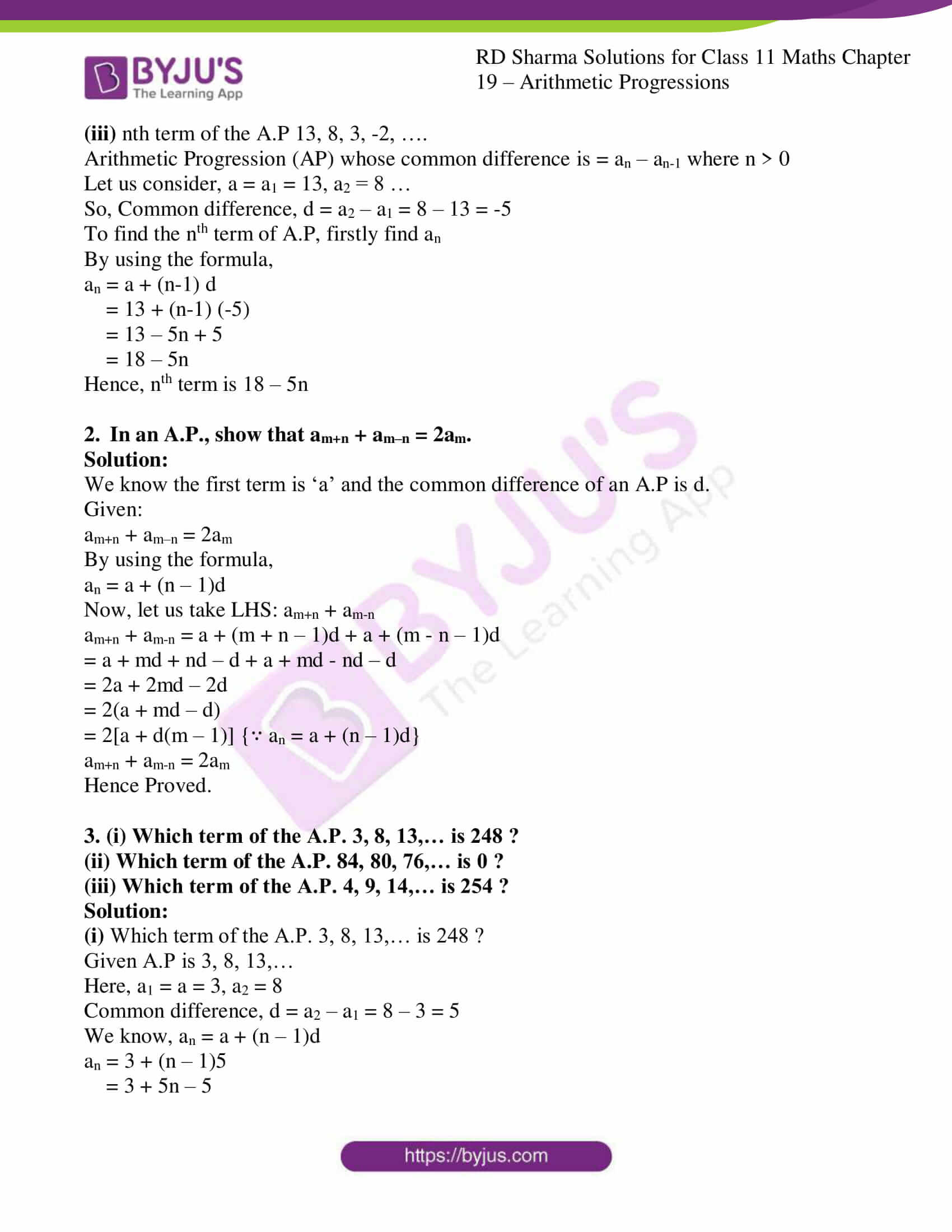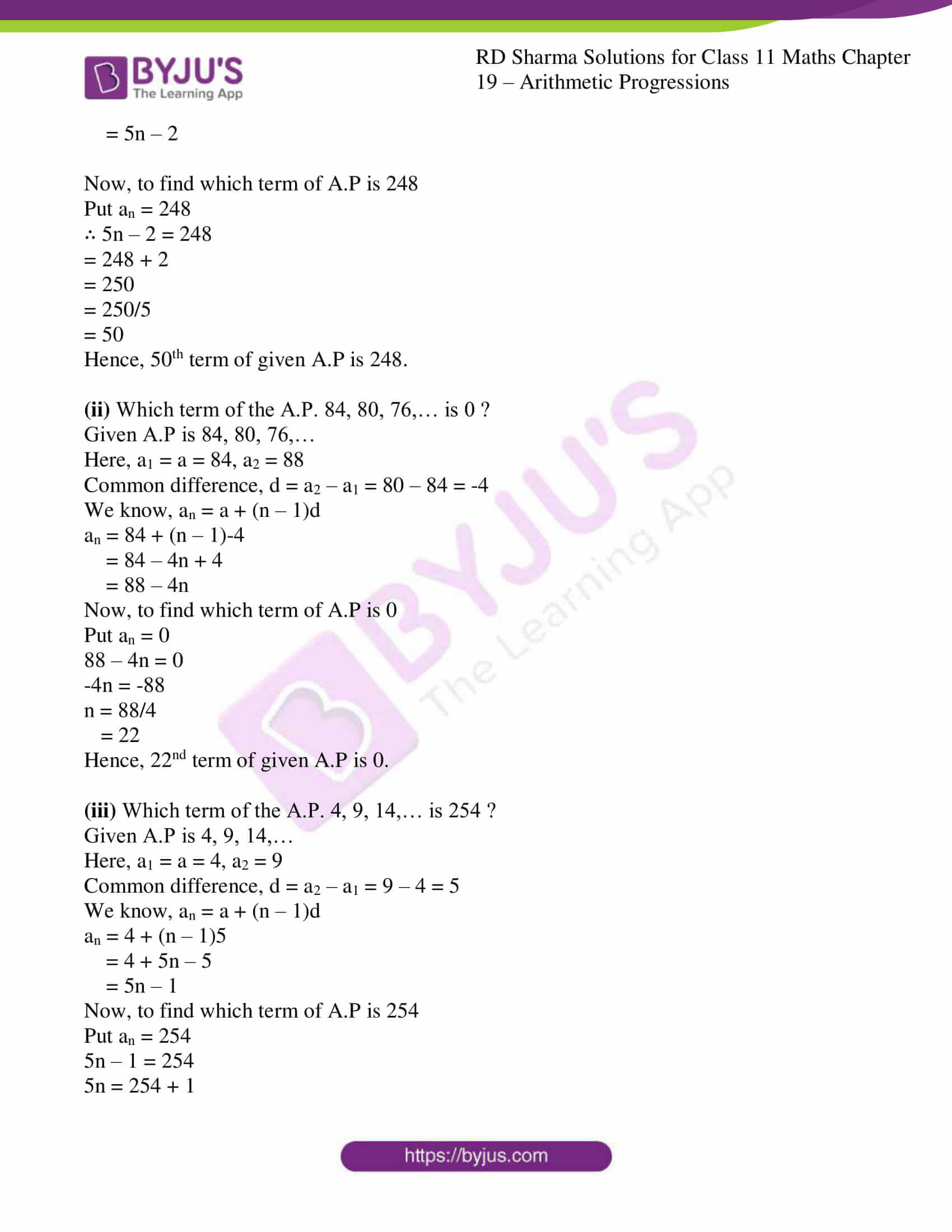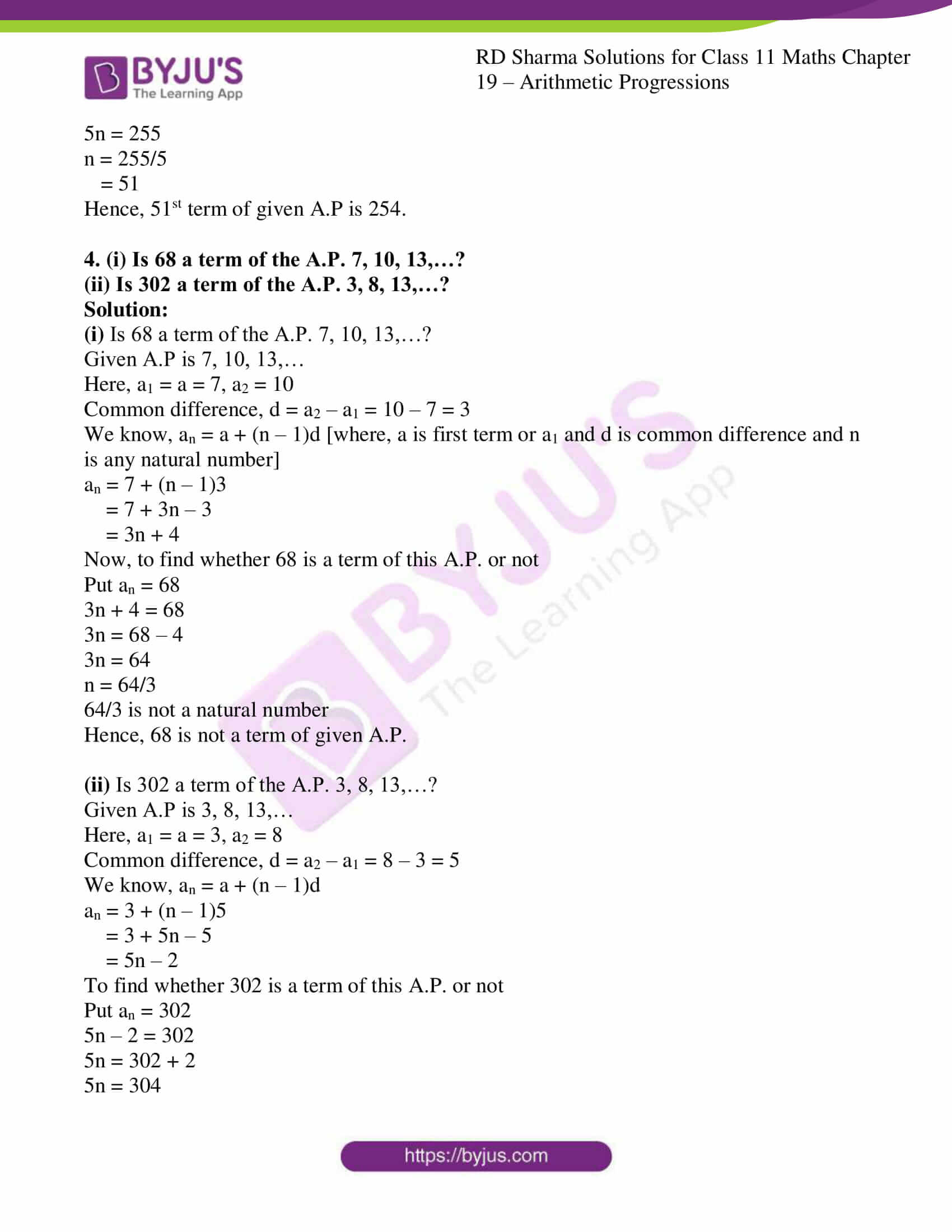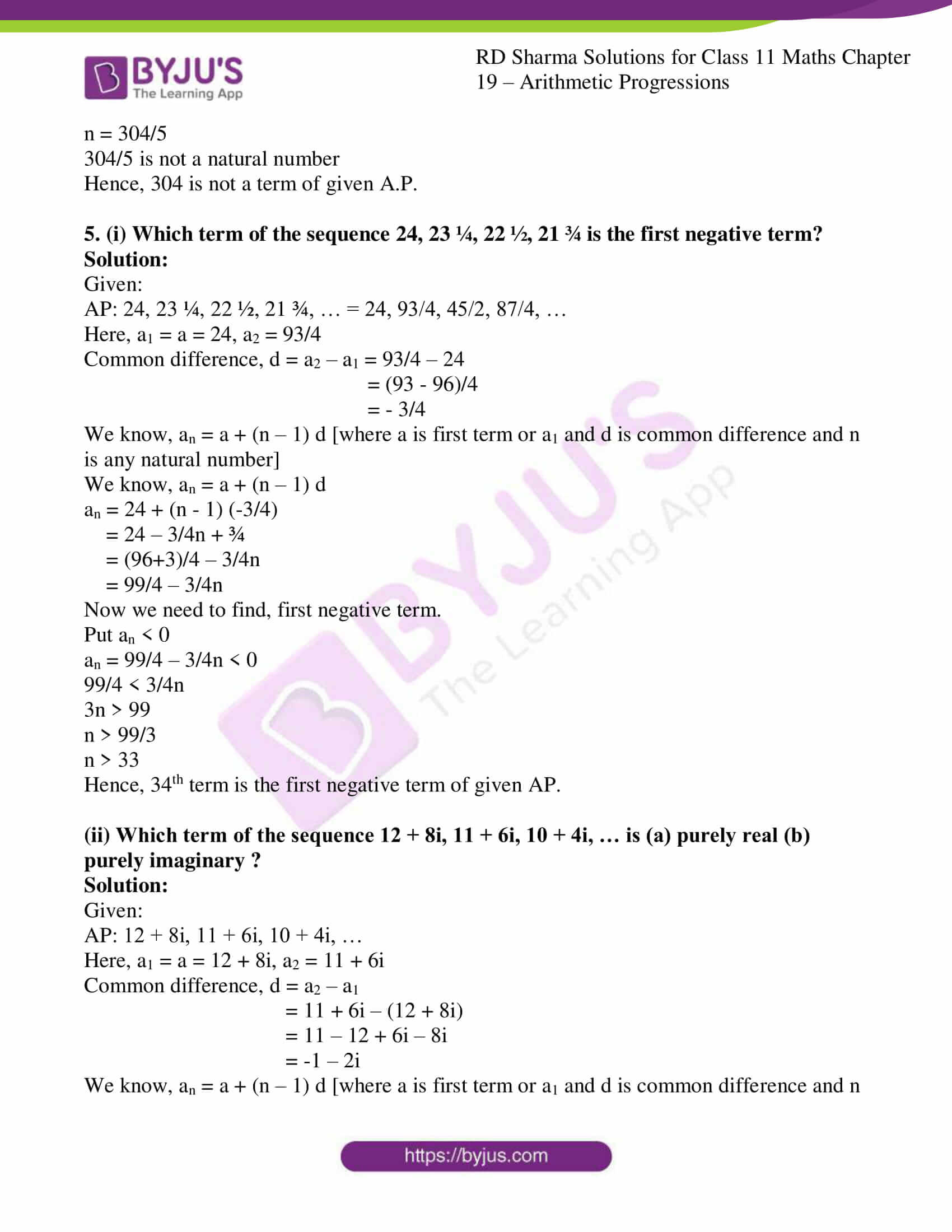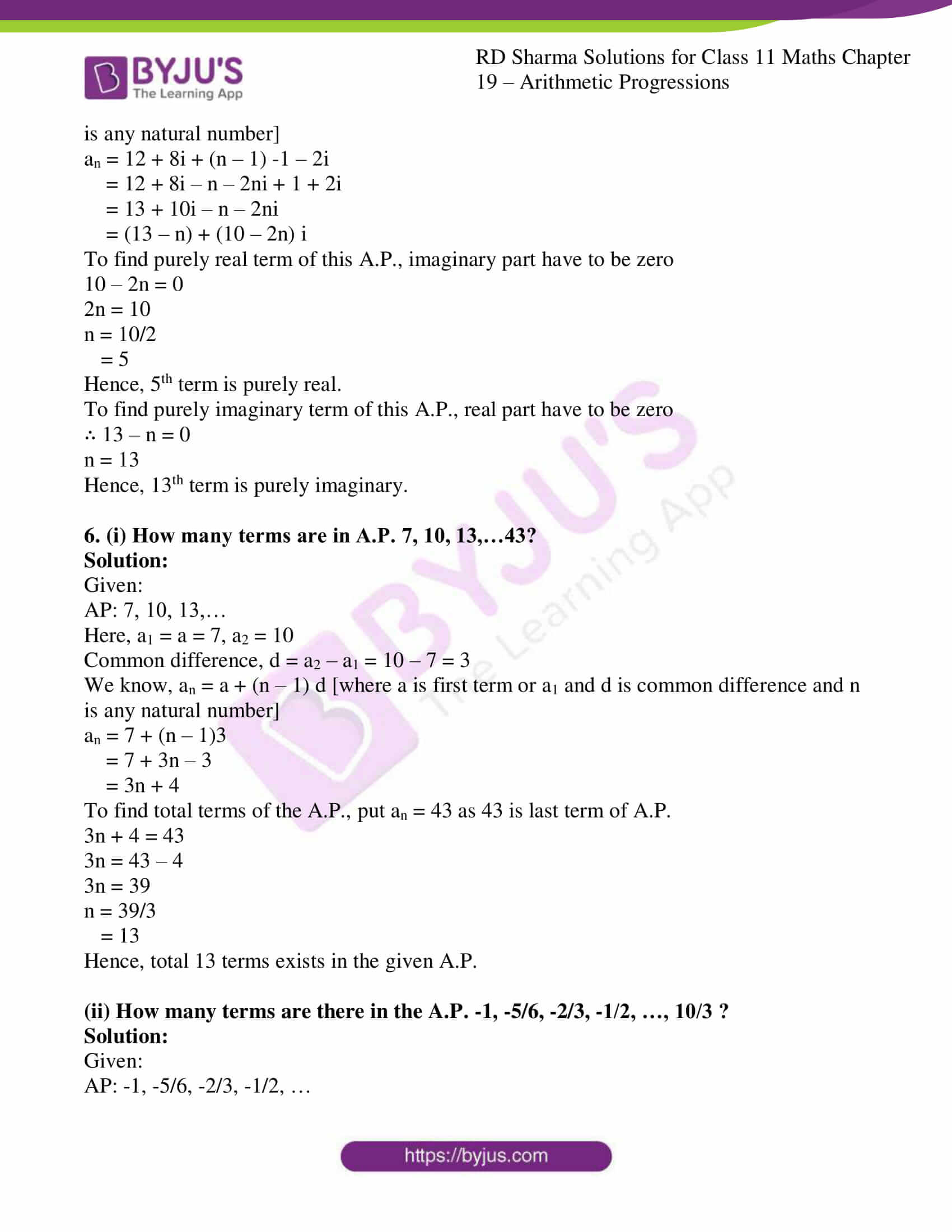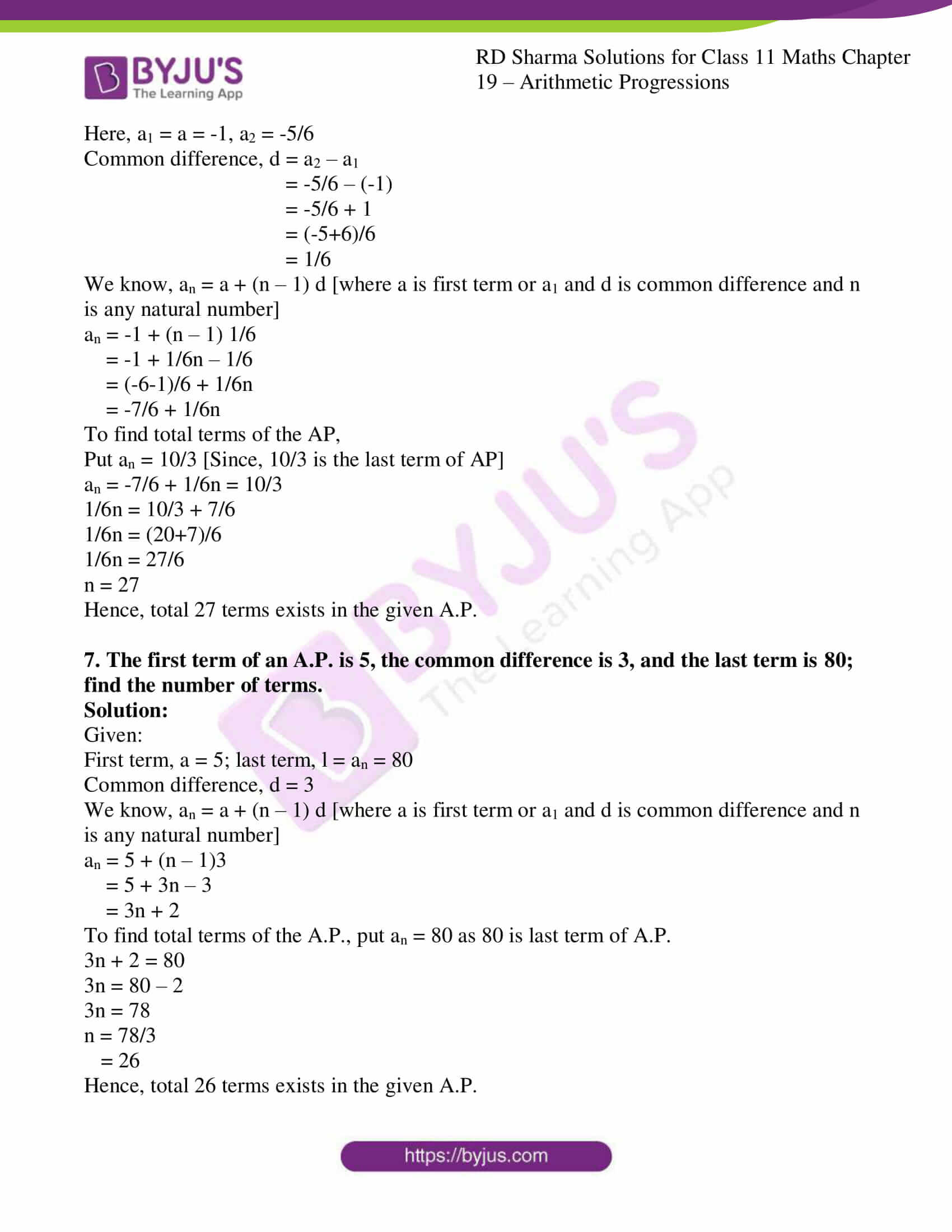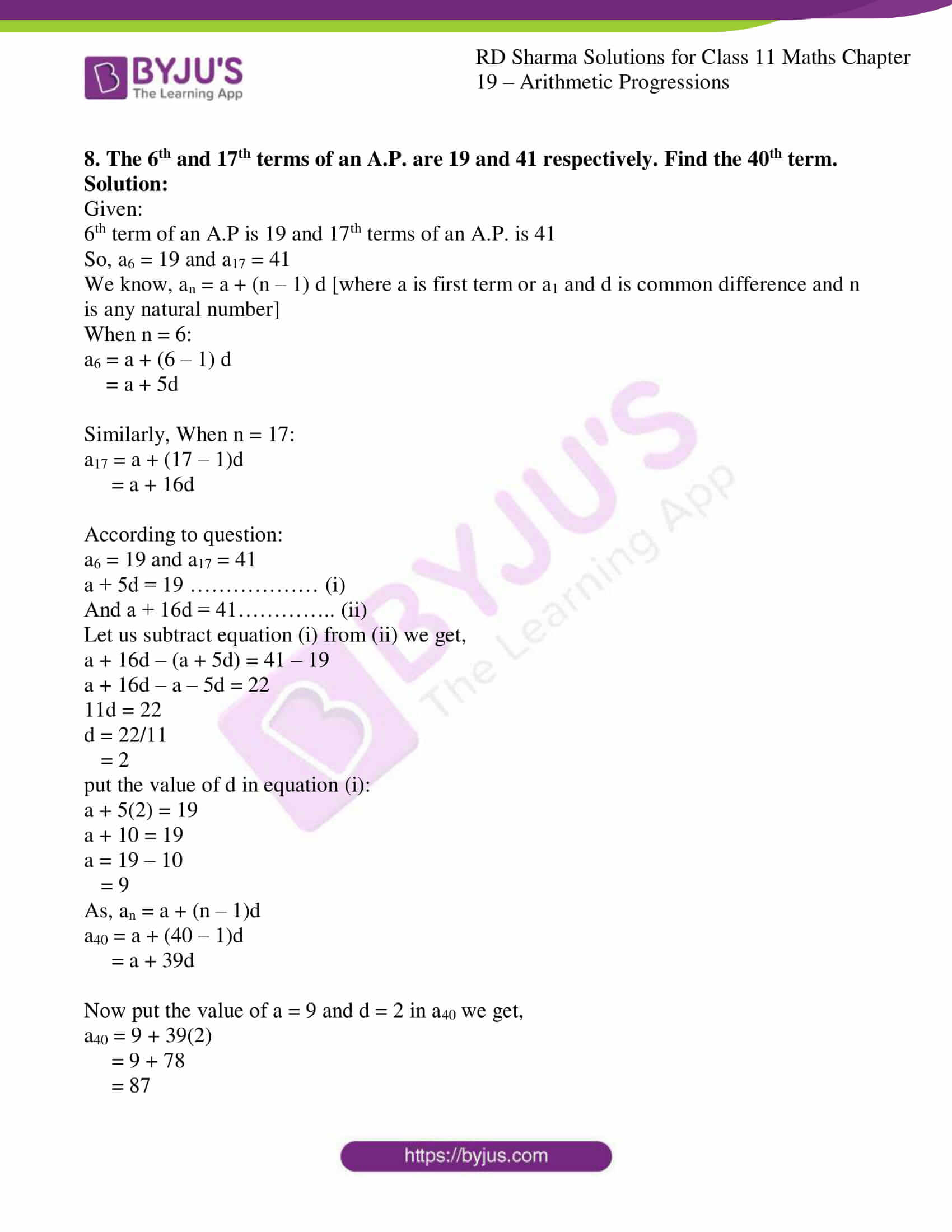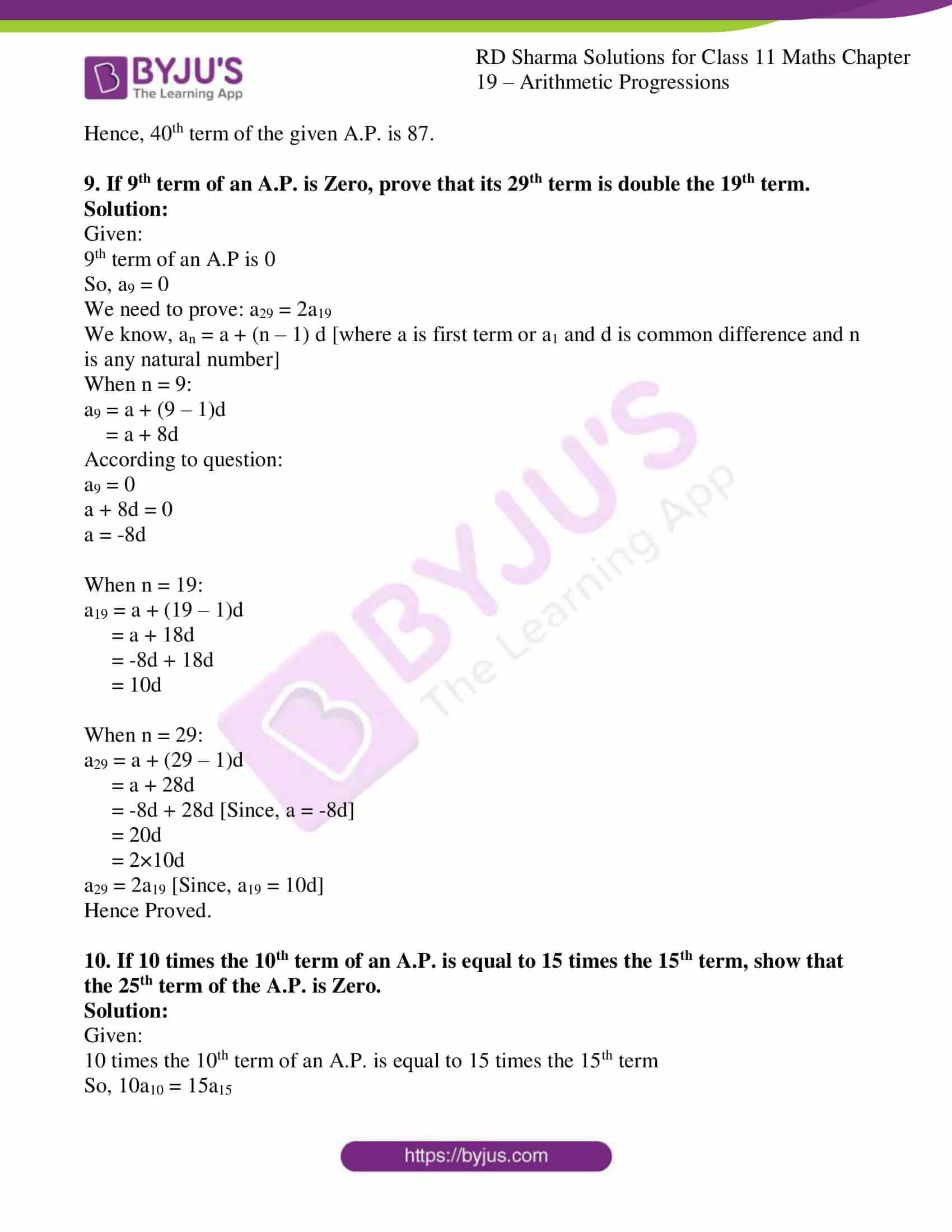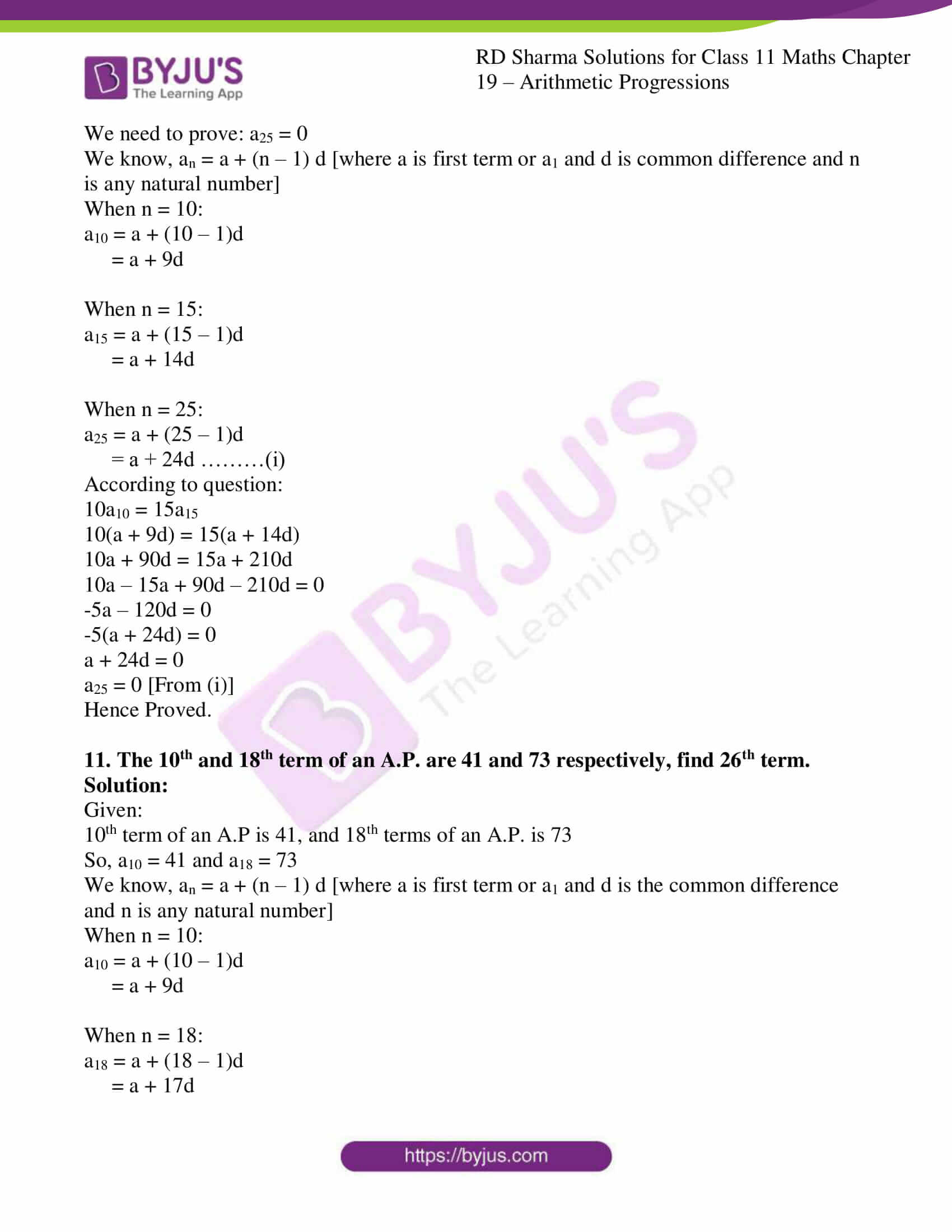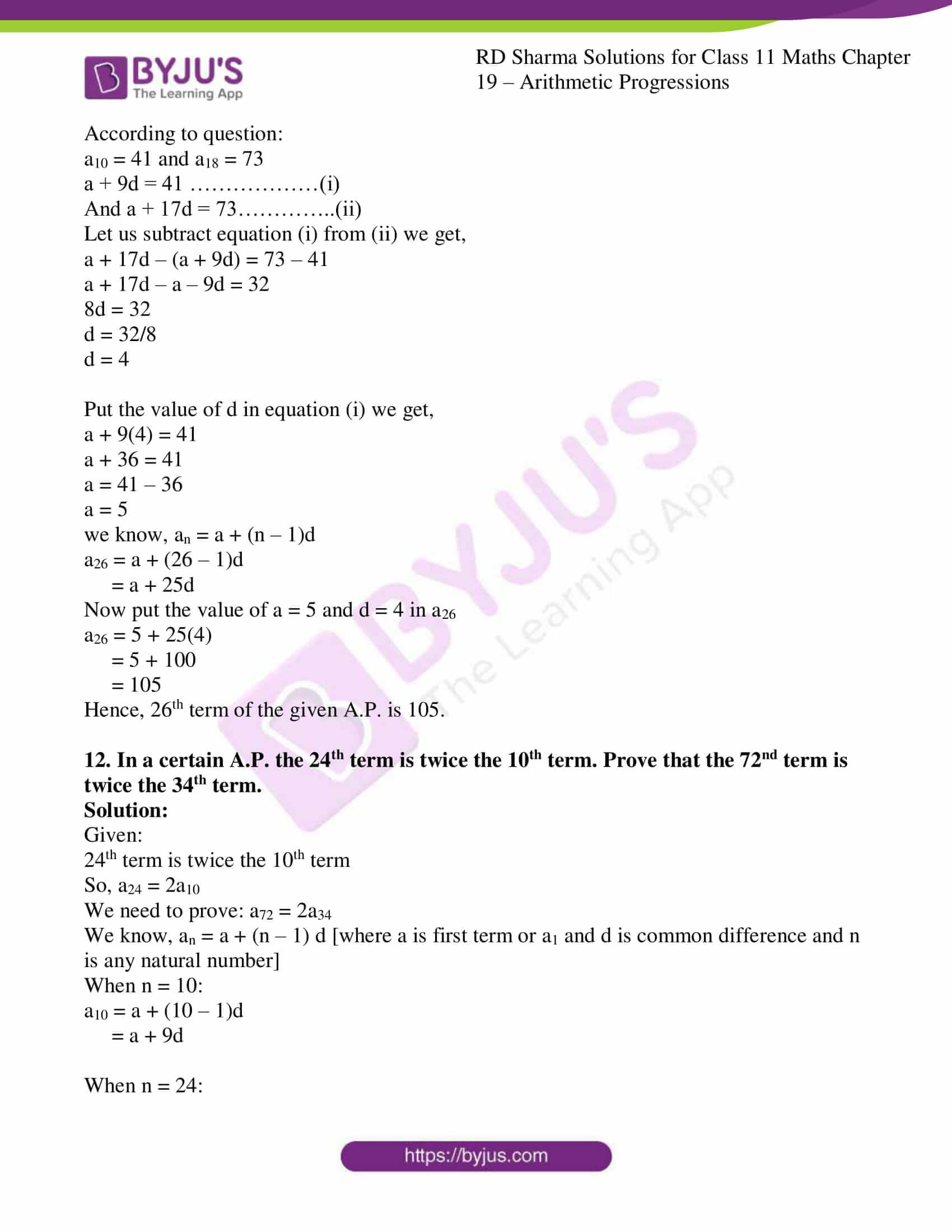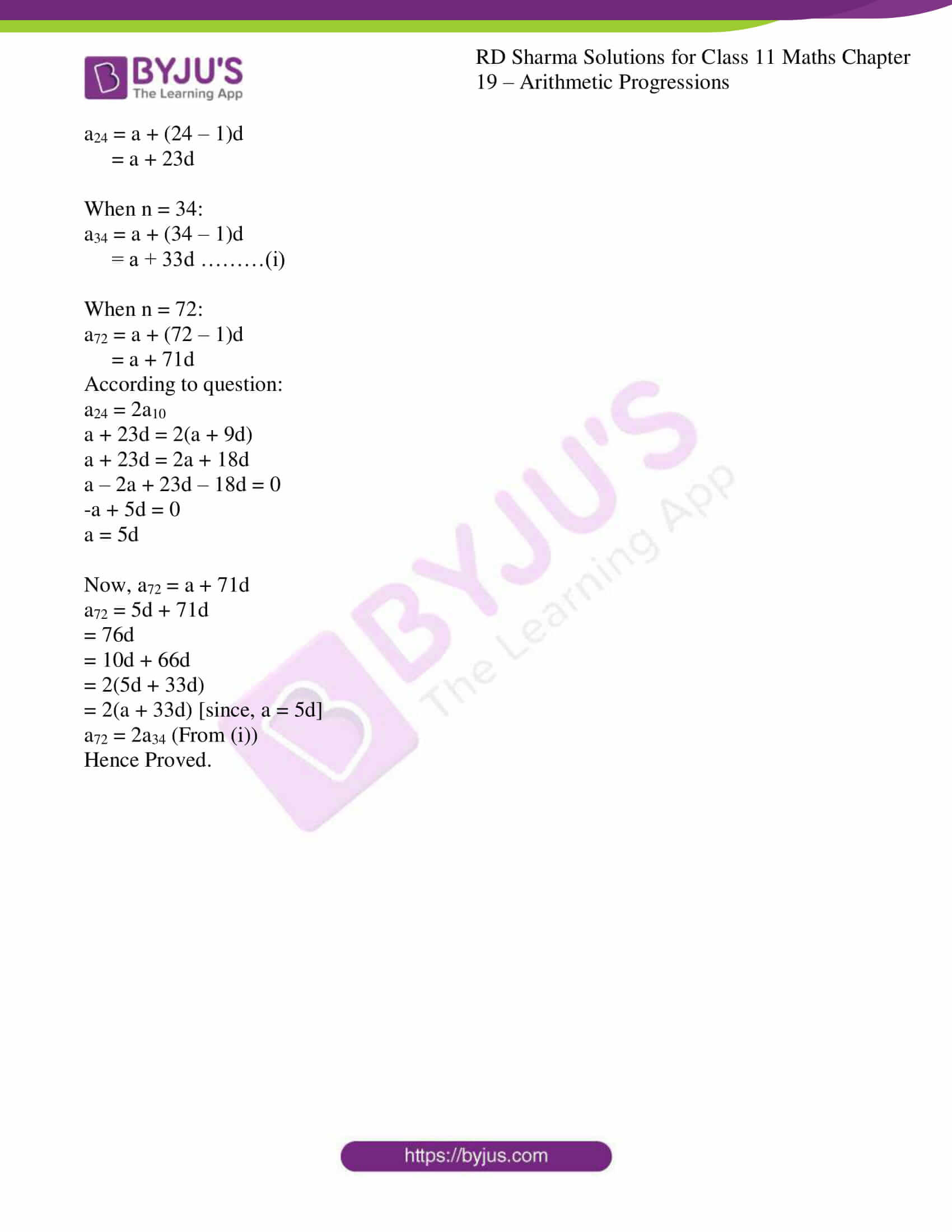### Also, access other exercises of RD Sharma Solutions for Class 11 Maths Chapter 19 – Arithmetic Progressions

Exercise 19.1 Solutions

Exercise 19.3 Solutions

Exercise 19.4 Solutions

Exercise 19.5 Solutions

Exercise 19.6 Solutions

Exercise 19.7 Solutions

### Access answers to RD Sharma Solutions for Class 11 Maths Exercise 19.2 Chapter 19 – Arithmetic Progressions

1. Find:
(i) 10th term of the A.P. 1, 4, 7, 10, …..

(ii) 18th term of the A.P. √2, 3√2, 5√2, …

(iii) nth term of the A.P 13, 8, 3, -2, ….

Solution:

(i) 10th term of the A.P. 1, 4, 7, 10, …..

Arithmetic Progression (AP) whose common difference is = an – an-1 where n > 0

Let us consider, a = a1 = 1, a2 = 4 …

So, Common difference, d = a2 – a1 = 4 – 1 = 3

To find the 10th term of A.P, firstly find an

By using the formula,

an = a + (n-1) d

= 1 + (n-1) 3

= 1 + 3n – 3

= 3n – 2

When n = 10:

a10 = 3(10) – 2

= 30 – 2

= 28

Hence, 10th term is 28.

(ii) 18th term of the A.P. √2, 3√2, 5√2, …

Arithmetic Progression (AP) whose common difference is = an – an-1 where n > 0

Let us consider, a = a1 = √2, a2 = 3√2 …

So, Common difference, d = a2 – a1 = 3√2 – √2 = 2√2

To find the 18th term of A.P, firstly find an

By using the formula,

an = a + (n-1) d

= √2 + (n – 1) 2√2

= √2 + 2√2n – 2√2

= 2√2n – √2

When n = 18:

a18 = 2√2(18) – √2

= 36√2 – √2

= 35√2

Hence, 10th term is 35√2

(iii) nth term of the A.P 13, 8, 3, -2, ….

Arithmetic Progression (AP) whose common difference is = an – an-1 where n > 0

Let us consider, a = a1 = 13, a2 = 8 …

So, Common difference, d = a2 – a1 = 8 – 13 = -5

To find the nth term of A.P, firstly find an

By using the formula,

an = a + (n-1) d

= 13 + (n-1) (-5)

= 13 – 5n + 5

= 18 – 5n

Hence, nth term is 18 – 5n

2. In an A.P., show that am+n + am–n = 2am.

Solution:

We know the first term is ‘a’ and the common difference of an A.P is d.

Given:

am+n + am–n = 2am

By using the formula,

an = a + (n – 1)d

Now, let us take LHS: am+n + am-n

am+n + am-n = a + (m + n – 1)d + a + (m – n – 1)d

= a + md + nd – d + a + md – nd – d

= 2a + 2md – 2d

= 2(a + md – d)

= 2[a + d(m – 1)] {∵ an = a + (n – 1)d}

am+n + am-n = 2am

Hence Proved.

3. (i) Which term of the A.P. 3, 8, 13,… is 248 ?

(ii) Which term of the A.P. 84, 80, 76,… is 0 ?

(iii) Which term of the A.P. 4, 9, 14,… is 254 ?

Solution:

(i) Which term of the A.P. 3, 8, 13,… is 248 ?

Given A.P is 3, 8, 13,…

Here, a1 = a = 3, a2 = 8

Common difference, d = a2 – a1 = 8 – 3 = 5

We know, an = a + (n – 1)d

an = 3 + (n – 1)5

= 3 + 5n – 5

= 5n – 2

Now, to find which term of A.P is 248

Put an = 248

∴ 5n – 2 = 248

= 248 + 2

= 250

= 250/5

= 50

Hence, 50th term of given A.P is 248.

(ii) Which term of the A.P. 84, 80, 76,… is 0 ?

Given A.P is 84, 80, 76,…

Here, a1 = a = 84, a2 = 88

Common difference, d = a2 – a1 = 80 – 84 = -4

We know, an = a + (n – 1)d

an = 84 + (n – 1)-4

= 84 – 4n + 4

= 88 – 4n

Now, to find which term of A.P is 0

Put an = 0

88 – 4n = 0

-4n = -88

n = 88/4

= 22

Hence, 22nd term of given A.P is 0.

(iii) Which term of the A.P. 4, 9, 14,… is 254 ?

Given A.P is 4, 9, 14,…

Here, a1 = a = 4, a2 = 9

Common difference, d = a2 – a1 = 9 – 4 = 5

We know, an = a + (n – 1)d

an = 4 + (n – 1)5

= 4 + 5n – 5

= 5n – 1

Now, to find which term of A.P is 254

Put an = 254

5n – 1 = 254

5n = 254 + 1

5n = 255

n = 255/5

= 51

Hence, 51st term of given A.P is 254.

4. (i) Is 68 a term of the A.P. 7, 10, 13,…?

(ii) Is 302 a term of the A.P. 3, 8, 13,…?

Solution:

(i) Is 68 a term of the A.P. 7, 10, 13,…?

Given A.P is 7, 10, 13,…

Here, a1 = a = 7, a2 = 10

Common difference, d = a2 – a1 = 10 – 7 = 3

We know, an = a + (n – 1)d [where, a is first term or a1 and d is common difference and n is any natural number]

an = 7 + (n – 1)3

= 7 + 3n – 3

= 3n + 4

Now, to find whether 68 is a term of this A.P. or not

Put an = 68

3n + 4 = 68

3n = 68 – 4

3n = 64

n = 64/3

64/3 is not a natural number

Hence, 68 is not a term of given A.P.

(ii) Is 302 a term of the A.P. 3, 8, 13,…?

Given A.P is 3, 8, 13,…

Here, a1 = a = 3, a2 = 8

Common difference, d = a2 – a1 = 8 – 3 = 5

We know, an = a + (n – 1)d

an = 3 + (n – 1)5

= 3 + 5n – 5

= 5n – 2

To find whether 302 is a term of this A.P. or not

Put an = 302

5n – 2 = 302

5n = 302 + 2

5n = 304

n = 304/5

304/5 is not a natural number

Hence, 304 is not a term of given A.P.

5. (i) Which term of the sequence 24, 23 ¼, 22 ½, 21 ¾ is the first negative term?

Solution:

Given:

AP: 24, 23 ¼, 22 ½, 21 ¾, … = 24, 93/4, 45/2, 87/4, …

Here, a1 = a = 24, a2 = 93/4

Common difference, d = a2 – a1 = 93/4 – 24

= (93 – 96)/4

= – 3/4

We know, an = a + (n – 1) d [where a is first term or a1 and d is common difference and n is any natural number]

We know, an = a + (n – 1) d

an = 24 + (n – 1) (-3/4)

= 24 – 3/4n + ¾

= (96+3)/4 – 3/4n

= 99/4 – 3/4n

Now we need to find, first negative term.

Put an < 0

an = 99/4 – 3/4n < 0

99/4 < 3/4n

3n > 99

n > 99/3

n > 33

Hence, 34th term is the first negative term of given AP.

(ii) Which term of the sequence 12 + 8i, 11 + 6i, 10 + 4i, … is (a) purely real (b) purely imaginary ?

Solution:

Given:

AP: 12 + 8i, 11 + 6i, 10 + 4i, …

Here, a1 = a = 12 + 8i, a2 = 11 + 6i

Common difference, d = a2 – a1

= 11 + 6i – (12 + 8i)

= 11 – 12 + 6i – 8i

= -1 – 2i

We know, an = a + (n – 1) d [where a is first term or a1 and d is common difference and n is any natural number]

an = 12 + 8i + (n – 1) -1 – 2i

= 12 + 8i – n – 2ni + 1 + 2i

= 13 + 10i – n – 2ni

= (13 – n) + (10 – 2n) i

To find purely real term of this A.P., imaginary part have to be zero

10 – 2n = 0

2n = 10

n = 10/2

= 5

Hence, 5th term is purely real.

To find purely imaginary term of this A.P., real part have to be zero

∴ 13 – n = 0

n = 13

Hence, 13th term is purely imaginary.

6. (i) How many terms are in A.P. 7, 10, 13,…43?

Solution:

Given:

AP: 7, 10, 13,…

Here, a1 = a = 7, a2 = 10

Common difference, d = a2 – a1 = 10 – 7 = 3

We know, an = a + (n – 1) d [where a is first term or a1 and d is common difference and n is any natural number]

an = 7 + (n – 1)3

= 7 + 3n – 3

= 3n + 4

To find total terms of the A.P., put an = 43 as 43 is last term of A.P.

3n + 4 = 43

3n = 43 – 4

3n = 39

n = 39/3

= 13

Hence, total 13 terms exists in the given A.P.

(ii) How many terms are there in the A.P. -1, -5/6, -2/3, -1/2, …, 10/3 ?

Solution:

Given:

AP: -1, -5/6, -2/3, -1/2, …

Here, a1 = a = -1, a2 = -5/6

Common difference, d = a2 – a1

= -5/6 – (-1)

= -5/6 + 1

= (-5+6)/6

= 1/6

We know, an = a + (n – 1) d [where a is first term or a1 and d is common difference and n is any natural number]

an = -1 + (n – 1) 1/6

= -1 + 1/6n – 1/6

= (-6-1)/6 + 1/6n

= -7/6 + 1/6n

To find total terms of the AP,

Put an = 10/3 [Since, 10/3 is the last term of AP]

an = -7/6 + 1/6n = 10/3

1/6n = 10/3 + 7/6

1/6n = (20+7)/6

1/6n = 27/6

n = 27

Hence, total 27 terms exists in the given A.P.

7. The first term of an A.P. is 5, the common difference is 3, and the last term is 80; find the number of terms.

Solution:

Given:

First term, a = 5; last term, l = an = 80

Common difference, d = 3

We know, an = a + (n – 1) d [where a is first term or a1 and d is common difference and n is any natural number]

an = 5 + (n – 1)3

= 5 + 3n – 3

= 3n + 2

To find total terms of the A.P., put an = 80 as 80 is last term of A.P.

3n + 2 = 80

3n = 80 – 2

3n = 78

n = 78/3

= 26

Hence, total 26 terms exists in the given A.P.

8. The 6th and 17th terms of an A.P. are 19 and 41 respectively. Find the 40th term.

Solution:

Given:

6th term of an A.P is 19 and 17th terms of an A.P. is 41

So, a6 = 19 and a17 = 41

We know, an = a + (n – 1) d [where a is first term or a1 and d is common difference and n is any natural number]

When n = 6:

a6 = a + (6 – 1) d

= a + 5d

Similarly, When n = 17:

a17 = a + (17 – 1)d

= a + 16d

According to question:

a6 = 19 and a17 = 41

a + 5d = 19 ……………… (i)

And a + 16d = 41………….. (ii)

Let us subtract equation (i) from (ii) we get,

a + 16d – (a + 5d) = 41 – 19

a + 16d – a – 5d = 22

11d = 22

d = 22/11

= 2

put the value of d in equation (i):

a + 5(2) = 19

a + 10 = 19

a = 19 – 10

= 9

As, an = a + (n – 1)d

a40 = a + (40 – 1)d

= a + 39d

Now put the value of a = 9 and d = 2 in a40 we get,

a40 = 9 + 39(2)

= 9 + 78

= 87

Hence, 40th term of the given A.P. is 87.

9. If 9th term of an A.P. is Zero, prove that its 29th term is double the 19th term.

Solution:

Given:

9th term of an A.P is 0

So, a9 = 0

We need to prove: a29 = 2a19

We know, an = a + (n – 1) d [where a is first term or a1 and d is common difference and n is any natural number]

When n = 9:

a9 = a + (9 – 1)d

= a + 8d

According to question:

a9 = 0

a + 8d = 0

a = -8d

When n = 19:

a19 = a + (19 – 1)d

= a + 18d

= -8d + 18d

= 10d

When n = 29:

a29 = a + (29 – 1)d

= a + 28d

= -8d + 28d [Since, a = -8d]

= 20d

= 2×10d

a29 = 2a19 [Since, a19 = 10d]

Hence Proved.

10. If 10 times the 10th term of an A.P. is equal to 15 times the 15th term, show that the 25th term of the A.P. is Zero.

Solution:

Given:

10 times the 10th term of an A.P. is equal to 15 times the 15th term

So, 10a10 = 15a15

We need to prove: a25 = 0

We know, an = a + (n – 1) d [where a is first term or a1 and d is common difference and n is any natural number]

When n = 10:

a10 = a + (10 – 1)d

= a + 9d

When n = 15:

a15 = a + (15 – 1)d

= a + 14d

When n = 25:

a25 = a + (25 – 1)d

= a + 24d ………(i)

According to question:

10a10 = 15a15

10(a + 9d) = 15(a + 14d)

10a + 90d = 15a + 210d

10a – 15a + 90d – 210d = 0

-5a – 120d = 0

-5(a + 24d) = 0

a + 24d = 0

a25 = 0 [From (i)]

Hence Proved.

11. The 10th and 18th term of an A.P. are 41 and 73 respectively, find 26th term.

Solution:

Given:

10th term of an A.P is 41, and 18th terms of an A.P. is 73

So, a10 = 41 and a18 = 73

We know, an = a + (n – 1) d [where a is first term or a1 and d is the common difference and n is any natural number]

When n = 10:

a10 = a + (10 – 1)d

= a + 9d

When n = 18:

a18 = a + (18 – 1)d

= a + 17d

According to question:

a10 = 41 and a18 = 73

a + 9d = 41 ………………(i)

And a + 17d = 73…………..(ii)

Let us subtract equation (i) from (ii) we get,

a + 17d – (a + 9d) = 73 – 41

a + 17d – a – 9d = 32

8d = 32

d = 32/8

d = 4

Put the value of d in equation (i) we get,

a + 9(4) = 41

a + 36 = 41

a = 41 – 36

a = 5

we know, an = a + (n – 1)d

a26 = a + (26 – 1)d

= a + 25d

Now put the value of a = 5 and d = 4 in a26

a26 = 5 + 25(4)

= 5 + 100

= 105

Hence, 26th term of the given A.P. is 105.

12. In a certain A.P. the 24th term is twice the 10th term. Prove that the 72nd term is twice the 34th term.

Solution:

Given:

24th term is twice the 10th term

So, a24 = 2a10

We need to prove: a72 = 2a34

We know, an = a + (n – 1) d [where a is first term or a1 and d is common difference and n is any natural number]

When n = 10:

a10 = a + (10 – 1)d

= a + 9d

When n = 24:

a24 = a + (24 – 1)d

= a + 23d

When n = 34:

a34 = a + (34 – 1)d

= a + 33d ………(i)

When n = 72:

a72 = a + (72 – 1)d

= a + 71d

According to question:

a24 = 2a10

a + 23d = 2(a + 9d)

a + 23d = 2a + 18d

a – 2a + 23d – 18d = 0

-a + 5d = 0

a = 5d

Now, a72 = a + 71d

a72 = 5d + 71d

= 76d

= 10d + 66d

= 2(5d + 33d)

= 2(a + 33d) [since, a = 5d]

a72 = 2a34 (From (i))

Hence Proved.